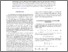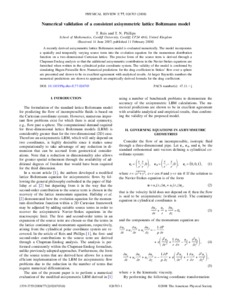# Numerical validation of a consistent axisymmetric lattice Boltzmann model

and Phillips, T. N. (2008) Numerical validation of a consistent axisymmetric lattice Boltzmann model. Physical Review E - Statistical, Nonlinear, and Soft Matter Physics, 77 (2):026703. ISSN 1539-3755 (Print), 1550-2376 (Online) (doi:https://doi.org/10.1103/PhysRevE.77.026703)Preview
PDF (Publisher's PDF)
23350 REIS_Numerical_Validation_of_a_Consistent_Axisymmetric_Lattice_Boltzmann_Model_2008.pdf - Published Version

## Abstract

A recently derived axisymmetric lattice Boltzmann model is evaluated numerically. The model incorporates a spatially and temporally varying source term into the evolution equation for the momentum distribution function on a two-dimensional Cartesian lattice. The precise form of the source term is derived through a Chapman-Enskog analysis so that the additional axisymmetric contributions in the Navier-Stokes equations are furnished when written in the cylindrical polar coordinate system. The validity of the model is confirmed by simulating Hagen-Poiseuille flow. Numerical predictions for the drag coefficient in Stokes’ flow over a sphere are presented and shown to be in excellent agreement with analytical results. At larger Reynolds numbers the numerical predictions are shown to approach an empirically derived formula for the drag coefficient.

Item Type: Article lattice Boltzmann, axisymmetric flow Q Science > QA Mathematics Faculty of Engineering & Science > School of Computing & Mathematical Sciences (CMS)Faculty of Engineering & Science 04 Mar 2022 13:08 http://gala.gre.ac.uk/id/eprint/23350View Item